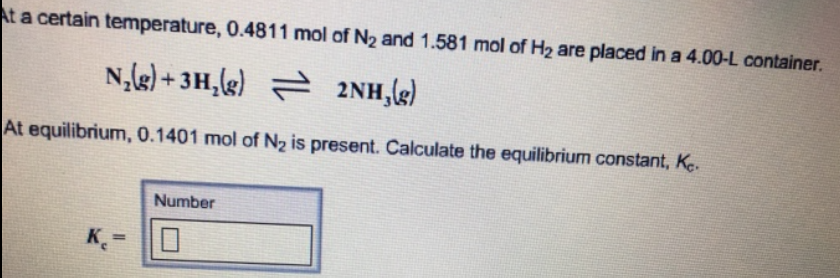# Problem: At a certain temperature, 0.4811 mol of N2 and 1.581 mol of H2 are placed in a 4.00-L container. N2 (g) + 3H2 (g) ⇌  2NH3 (g) At equilibrium, 0.1401 mol of N2 is present. Calculate the equilibrium constant, Kc.

###### FREE Expert Solution
83% (51 ratings)###### Problem Details

At a certain temperature, 0.4811 mol of N2 and 1.581 mol of H2 are placed in a 4.00-L container.

N2 (g) + 3H2 (g) ⇌  2NH3 (g)

At equilibrium, 0.1401 mol of N2 is present. Calculate the equilibrium constant, Kc.